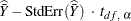### Confidence Limits for the Mean

If you specify the keyword CLM, the procedure computes two-sided confidence limits for the mean. Also, the procedure includes the confidence limits by default if you do not specify any statistic-keywords in the PROC SURVEYMEANS  statement.

The confidence coefficient is determined by the value of the ALPHA= option, which by default equals 0.05 and produces 95% confidence limits. The confidence limits are computed as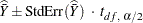where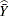is the estimate of the mean,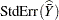is the standard error of the mean, and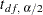is the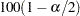th percentile of the t distribution with df calculated as described in the section t Test for the Mean.

If you specify the keyword UCLM, the procedure computes the one-sided upper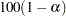% confidence limit for the mean: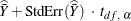If you specify the keyword LCLM, the procedure computes the one-sided lower% confidence limit for the mean: